Home | Study Links | Rhymes |

## Squaring the Circle - 396 x 8 = 3168

While working on the age-old “Squaring the Circle” challenge, I discovered a construction
method that produced a very accurate result. The challenge is as accurate as one can be,
to construct a square with the same area as that of a given circle, or visa versa, using only
a pair of compasses for drawing circles and a ruler for drawing straight lines.

I experimented with the interesting numbers 3-6-9, and 396 became the diameter for a circle.
Of course, now that the technique is known, any circle diameter will do.
The square area in my experiment: (a-b 2772 mm x 2) 2 = 30735936.
My circle radius result: 3127.86697555..., with an area of 30735934.51364089...
I managed an accuracy of at least 99.999995... %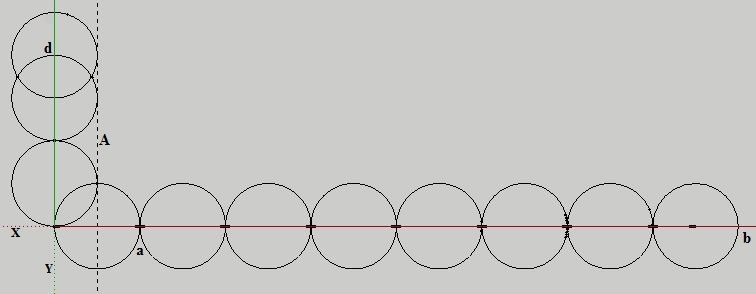Place 8 circles along the X-axis and 2.5 on the Y-axis, and add guide-line A.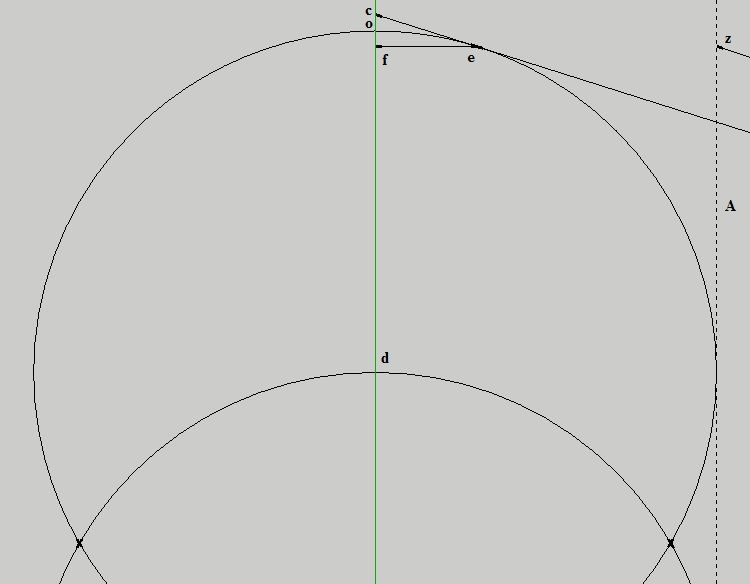Connect a line starting from b (diagram 1) to c (on the Y-axis), touching the circle at "e"
as accurately as possible. Important! The accuracy of the final result depends on it.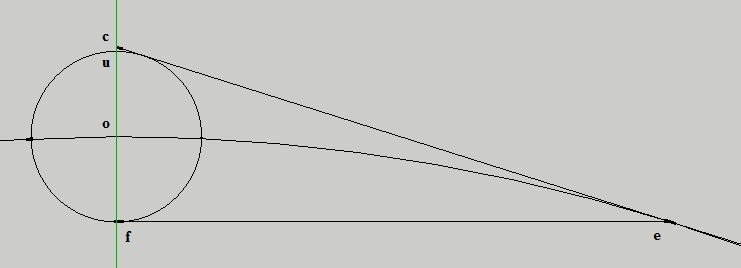Draw the line e-f parallel to the X-axis, and circle radius o-f.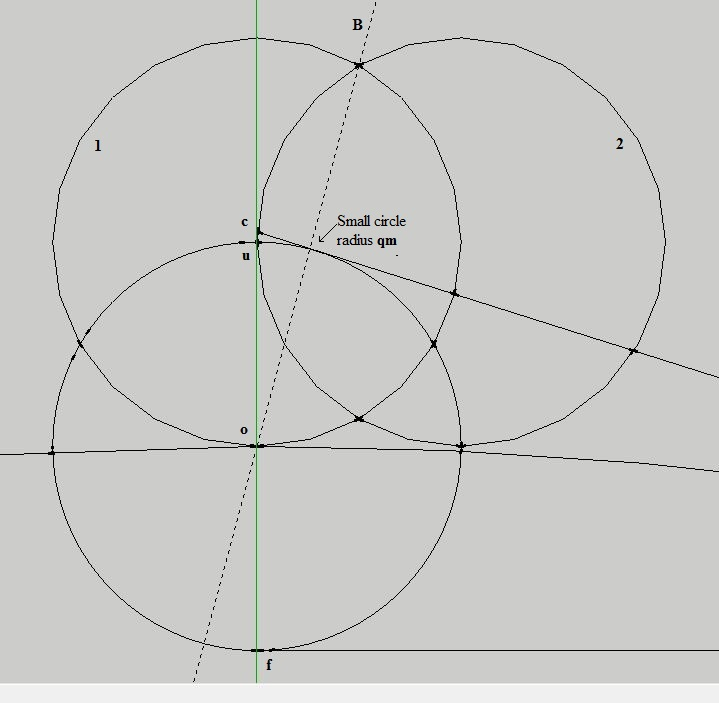Draw circle 1 (radius u-o), then 2, then add guide line B.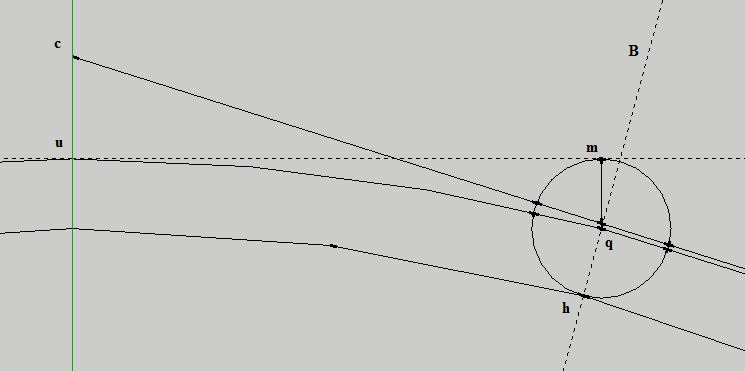Circle radius q-m as shown (q = B guide line and circle). It produces point h, and the inner circle.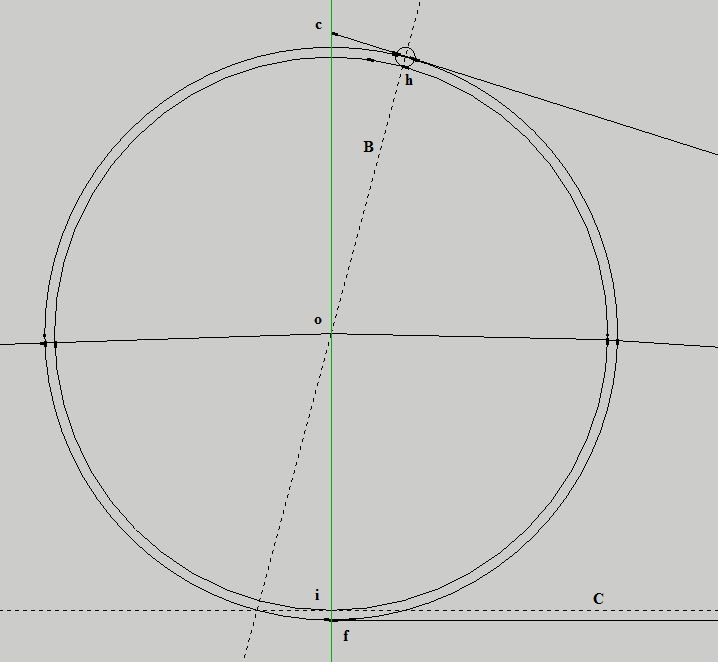Circle radius o-h and draw guide line C through point i.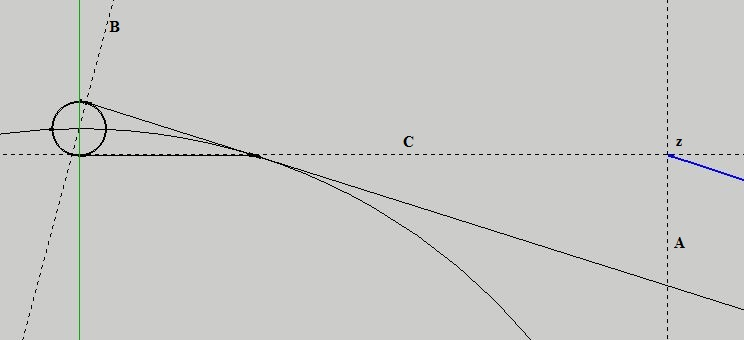Intersection of guide lines C and A is z.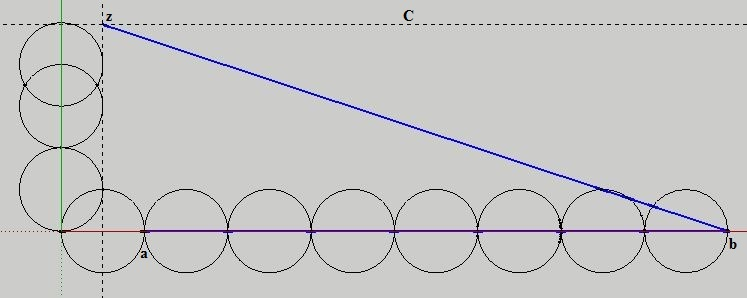Draw line b-z = the circle radius.
Circle area (radius b-z) 2 x π should closely match Square area (line a-b x 2) 2

Doing this by compass and pencil one can get a quite accurate result,
by drawing the C guide line just below the top of the upper circle.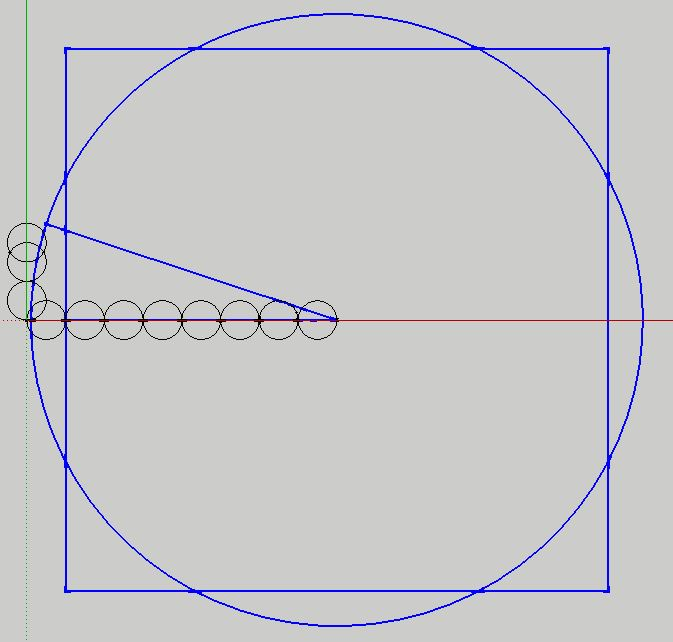There are a few variation possibilities to this construction method.

><><

Lastly, a less accurate but easier construction method where (A-D)2 + (D-B)2 = (A-B)2 :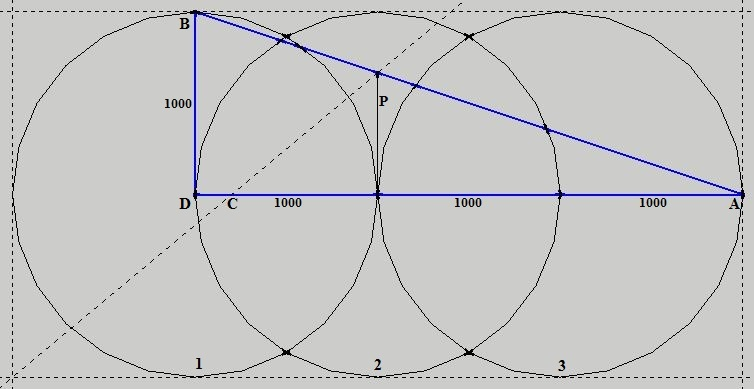1. Draw circles 1, 2, 3
2. Draw triangle and post.
3. Draw guide lines as shown.

Circle radius A-B = √10 000 000 mm. Area = π x 10 000 000 (31415926.535... mm)

A-C = 2800 mm, and a square area of (2800 x 2) 2 = 31360000 mm

Accurate to 99.9%